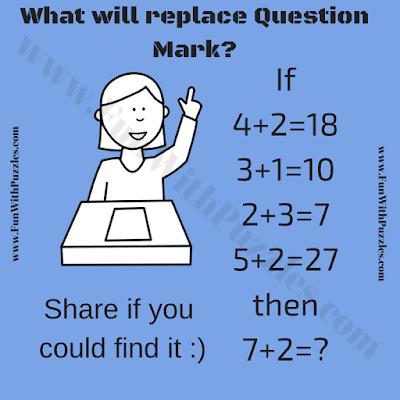How quick can you think logically? Here is the Logical Maths Question to test your logical reasoning and Mathematical skill. In this Logical Maths Question, there are some logical equations. Your challenge is to find the logical reasoning for these Logical Maths Equations and then solve the last equation. Can you solve this Logical Maths Question?Can you find the missing number?

The answer to this "Logical Maths Question", can be viewed by clicking on the button. Please do give your best try before looking at the answer.

Unknown said...

Ans 16

Unknown said...

51

Unknown said...

I have no idea... ummm 16?

Unknown said...

Ans is 7

Anonymous said...

The answer is 51 now I’ll give you an explanation so the first one was 4+2=18 I just took away 18-2 and that was 16 4×4 is 16 then the next one is 3+1 equals 10 10 minus one is 9 3×3 is nine and 5+2+27 27-2= 25. 5×5=25 then is obviously we do 7+2 = 51 51-2=49 7x7 = 49 so it’s 51

Unknown said...

Answer is 51 because in the question we have 4+2=18 which means square of 4 which is 16 + 2 =18 then 3 + 1 = 10 which means square of 3 which 9+1=10
2+3=7 which means square of 2 which is 4+3=7, 5+2=27 which means square of 5 which is 25+2=27, as same with 7+2=? Square of seven which is 49+2=51